### Measurements Class 8th Mathematics Term 1 Tamilnadu Board Solution

##### Question 1.Find the perimeter of the following figures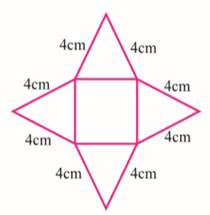Answer:Perimeter = 4 + 4 + 4 + 4 + 4 + 4 + 4 + 4= 32 cmQuestion 2.Find the perimeter of the following figures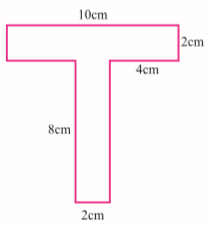Answer:Perimeter = 10 + 2 + 4 + 8 + 2 + 8 + 4 + 2= 40 cmQuestion 3.Find the perimeter of the following figures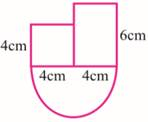Answer:Radius of semi-circle = 4 cmPerimeter of semi-circle = πr= 3.14 × 4= 12.56 cmPerimeter of figure = 12.56 + 4 + 4 + (6-4) + 4 + 6= 30.56 cmQuestion 4.Find the perimeter of the following figures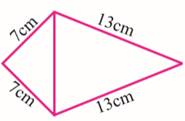Answer:Perimeter = 7 + 13 + 13 + 7= 40 cmQuestion 5.Find the perimeter of the following figures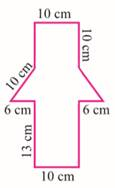Answer:Perimeter = 10 + 10 + 10 + 6 + 13 + 10 + 13 + 6 + 10 + 10= 98 cmQuestion 6.Find the area of the following figures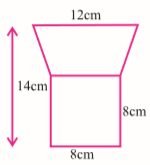Answer:Height of trapezium = 14-8= 6 cmArea of trapezium is given as, A = 1/2 × (a + b)h,As shown below: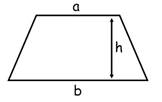Where, a is the shorter side.B is the longer side.H is the distance between the two sides.⇒ Area of trapezium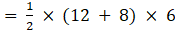= 60 cm2Area of square = 8 × 8= 64 cm2Area of figure = Area of trapezium + Area of square= 60 + 64= 124 cm2Question 7.Find the area of the following figures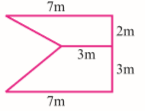Answer:The figure can be re-drawn as: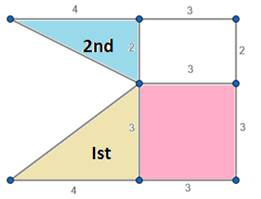Area of first triangle = 1/2 × base × height⇒ A1 =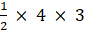= 6 cm2Area of second triangle = 1/2 × base × height⇒ A2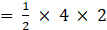= 4 cm2Area of rectangle = length × breadth⇒ A3 = 3 × 2= 6 cm2Area of square = (side)2⇒ A4 = 3 × 3= 9 cm2∴ Area of figure = A1 + A2 + A3 + A4= 6 + 4 + 6 + 9= 25 cm2Question 8.Find the area of the following figures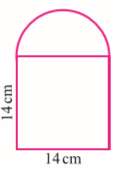Answer:Diameter of semicircle = 14cmRadius of semicircle =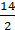= 7 cm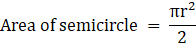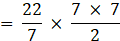= 77 cm2Area of square = (side)2 = 14 × 14= 196 cm2Area of figure = Area of semicircle + Area of square= 77 + 196= 273 cm2Question 9.Find the area of the following figures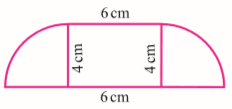Answer:We know,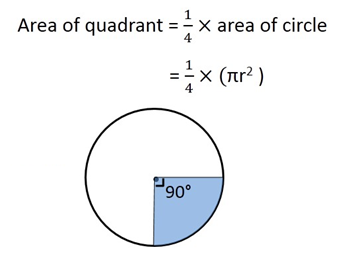Area of two quadrants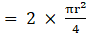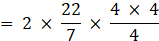= 25.14 cm2Area of rectangle = length × breadth= 6 × 4= 24 cm2Question 10.Find the area of the following figures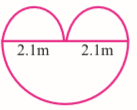Answer:Radius of bigger semicircle = 2.1 mRadius of smaller semicircles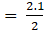= 1.05 mArea of 2 smaller semicircles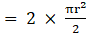∴Area of 2 smaller semicircles = πr2Hence, area of 2 smaller semicircles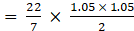= 1.7325 m2Area of bigger semicircle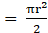∴Area of bigger semicircle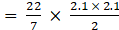= 6.93 m2Question 11.Find the area of the coloured regions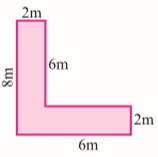Answer:The figure is given below: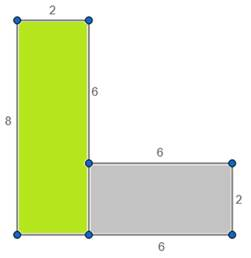Area of bigger rectangle (shaded in green) = length × breadth= 8 × 2= 16 m2Area of smaller rectangle (shaded in grey) = length × breadth= 6 × 2= 12 m2Area of the coloured regions = Area of bigger rectangle + Area ofsmaller rectangleArea of the coloured regions = 16 + 12= 28 m2Question 12.Find the area of the coloured regions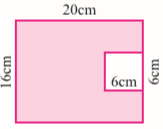Answer:Area of rectangle = length × breadth= 16 × 20= 320 m2Area of square = side × side= 6 × 6= 36 m2Area of the coloured regions = Area of rectangle + Area of squareArea of the coloured regions = 320 + 36= 356 m2Question 13.Find the area of the coloured regions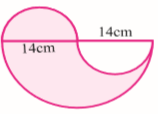Answer:Radius of smaller semicircle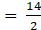= 7 cmRadius of bigger semicircle = 14 cmArea of smaller semicircle= 77 cm2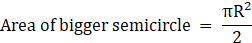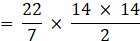= 308 cm2Area of the coloured regions = (Area of bigger semicircle-Area ofsmaller semicircle) + Area of smaller semicircleArea of the coloured regions = (308-77) + 77= 308 cm2Question 14.Find the area of the coloured regions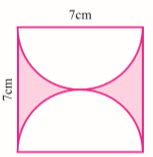Answer:Area of square = 7 × 7= 49 cm2Area of semicircle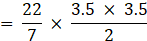= 19.25 cm2Area of coloured region = Area of square - 2 × Area of semicircle= 49-2 × 19.25= 49-38.5= 10.5 cm2Question 15.Find the area of the coloured regions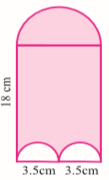Answer:Area of rectangle = 18 × 7= 126 cm2Radius of bigger semicircle = 3.5 cmArea of bigger semicircle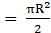= 19.25 cm2Radius of smaller semicircle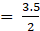= 1.75 cmArea of unshaded region = πr2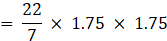= 9.625 cm2Area of coloured region = Area of bigger semicircle + ( Area ofRectangle- Area of unshaded region)Area of coloured region = 19.25 + (126-9.625)= 19.25 + 116.375= 135.625 cm2Question 16.Find the area of the coloured regions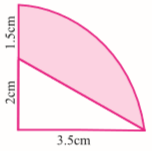Answer: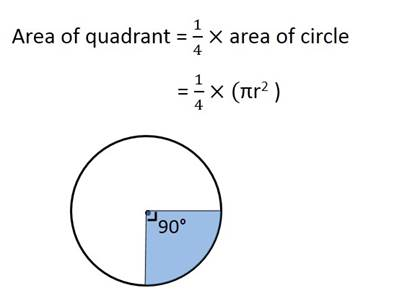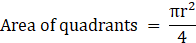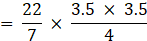= 9.625 cm2Area of triangle = 1/2 × base × height= 1/2 × 3.5 × 2= 3.5 cm2Area of coloured region = Area of quadrant - Area of triangle= 9.625-3.5= 6.125 cm2Question 17.In the given figure, find the area of the shaded portion if AC = 54 cm, BC = 10 cm, and O is the centre of bigger circle.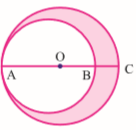Answer:Given, AC = 54 cmBC = 10 cmAB = 54-10 = 44 cmRadius of bigger circle =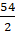= 27 cmArea of bigger circle = πr2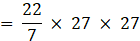= 2291.14Radius of smaller circle =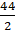= 22 cmArea of smaller circle = πR2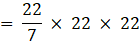= 1521.14Area of the shaded portion = Area of bigger circle- Area of smallerCircle= 2291.14-1521.14= 769.99 cm2= 770 cm2Question 18.A cow is tied up for grazing inside a rectangular field of dimensions 40 m × 36 m in one corner of the field by a rope of length 14 m. Find the area of the field left ungrazed by the cow.Answer:The figure is shown below: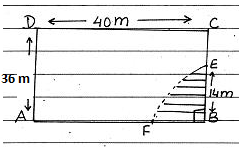Area of rectangular field = 40 × 36= 1440 m2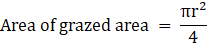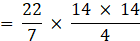= 154 m2Therefore, Area of the field left ungrazed by the cow = 1440-154= 1286 m2Question 19.A square park has each side of 100 m. At each corner of the park there is a flower bed in the form of a quadrant of radius 14 m as shown in the figure. Find the area of the remaining portion of the park.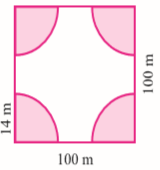Answer:Radius = 14 cmOne flower bed is a quadrant of the circle.We know,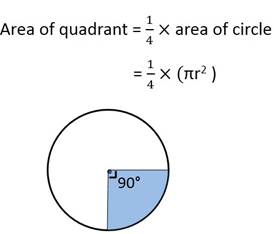⇒ Area of one flower bed = 3.14 × 14 × 14= 616 m2Area of the square park = 100 × 100= 10000 m2Area of the four-flower bed = 4 × 616= 2464 m2Thus area of the remaining part = (10000-2464) m2= 7536 m2Question 20.Find the area of the shaded region shown in the figure. The four corners are quadrants. At the center, there is a circle of diameter 2 cm.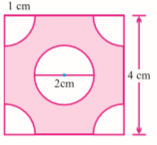Answer:Area of square = side × side= 4 × 4= 16 cm2Area of unshaded region = 4 × Area of 1 quadrant + Area of circle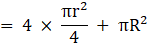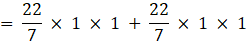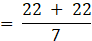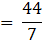= 6.28 cm2Therefore,Area of shaded region = Area of square- Area ofunshaded regionArea of shaded region = 16-6.28= 9.72 cm2Question 21.A paper is in the form of a rectangle ABCD in which AB = 20 cm and BC = 14 cm. A semicircular portion with BC as diameter is cut off. Find the area of the remaining part.Answer:The figure is given below: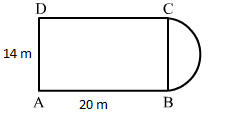Diameter of semi-circle = BC = 14cmRadius of semi–circle= 7cmArea of semi-circle= 77 cm2Area of sheet = 20 × 14 = 280 cm2Thus, Area of remaining sheet = 280-77= 203 cm2Question 22.On a square handkerchief, nine circular designs each of radius 7 cm are made. Find the area of the remaining portion of the handkerchief.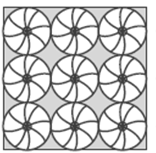Answer:From the figure,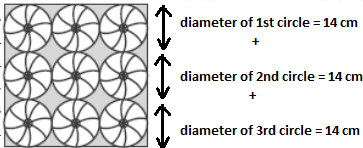Hence, it can be observed that size of side of square = 14 + 14 + 14 = 42 cmArea of square = (side)2= 42 × 42= 1764 cm2Area of each circle = πr2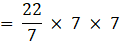= 154 cm2Area of 9 circles = 9 × 154= 1386 cm2Area of unshaded region = Area of square – Area of 9 circle= 1764 -1386= 378 cm2

PDF FILE TO YOUR EMAIL IMMEDIATELY PURCHASE NOTES & PAPER SOLUTION. @ Rs. 50/- each (GST extra)

HINDI ENTIRE PAPER SOLUTION

MARATHI PAPER SOLUTION

SSC MATHS I PAPER SOLUTION

SSC MATHS II PAPER SOLUTION

SSC SCIENCE I PAPER SOLUTION

SSC SCIENCE II PAPER SOLUTION

SSC ENGLISH PAPER SOLUTION

SSC & HSC ENGLISH WRITING SKILL

HSC ACCOUNTS NOTES

HSC OCM NOTES

HSC ECONOMICS NOTES

HSC SECRETARIAL PRACTICE NOTES

# 2019 Board Paper Solution

HSC ENGLISH SET A 2019 21st February, 2019

HSC ENGLISH SET B 2019 21st February, 2019

HSC ENGLISH SET C 2019 21st February, 2019

HSC ENGLISH SET D 2019 21st February, 2019

SECRETARIAL PRACTICE (S.P) 2019 25th February, 2019

HSC XII PHYSICS 2019 25th February, 2019

CHEMISTRY XII HSC SOLUTION 27th, February, 2019

OCM PAPER SOLUTION 2019 27th, February, 2019

HSC MATHS PAPER SOLUTION COMMERCE, 2nd March, 2019

HSC MATHS PAPER SOLUTION SCIENCE 2nd, March, 2019

SSC ENGLISH STD 10 5TH MARCH, 2019.

HSC XII ACCOUNTS 2019 6th March, 2019

HSC XII BIOLOGY 2019 6TH March, 2019

HSC XII ECONOMICS 9Th March 2019

SSC Maths I March 2019 Solution 10th Standard11th, March, 2019

SSC MATHS II MARCH 2019 SOLUTION 10TH STD.13th March, 2019

SSC SCIENCE I MARCH 2019 SOLUTION 10TH STD. 15th March, 2019.

SSC SCIENCE II MARCH 2019 SOLUTION 10TH STD. 18th March, 2019.

SSC SOCIAL SCIENCE I MARCH 2019 SOLUTION20th March, 2019

SSC SOCIAL SCIENCE II MARCH 2019 SOLUTION, 22nd March, 2019

XII CBSE - BOARD - MARCH - 2019 ENGLISH - QP + SOLUTIONS, 2nd March, 2019

# HSCMaharashtraBoardPapers2020

(Std 12th English Medium)

HSC ECONOMICS MARCH 2020

HSC OCM MARCH 2020

HSC ACCOUNTS MARCH 2020

HSC S.P. MARCH 2020

HSC ENGLISH MARCH 2020

HSC HINDI MARCH 2020

HSC MARATHI MARCH 2020

HSC MATHS MARCH 2020

# SSCMaharashtraBoardPapers2020

(Std 10th English Medium)

English MARCH 2020

HindI MARCH 2020

Hindi (Composite) MARCH 2020

Marathi MARCH 2020

Mathematics (Paper 1) MARCH 2020

Mathematics (Paper 2) MARCH 2020

Sanskrit MARCH 2020

Sanskrit (Composite) MARCH 2020

Science (Paper 1) MARCH 2020

Science (Paper 2)

Geography Model Set 1 2020-2021

MUST REMEMBER THINGS on the day of Exam

Are you prepared? for English Grammar in Board Exam.

Paper Presentation In Board Exam

How to Score Good Marks in SSC Board Exams

Tips To Score More Than 90% Marks In 12th Board Exam

How to write English exams?

How to prepare for board exam when less time is left

How to memorise what you learn for board exam

No. 1 Simple Hack, you can try out, in preparing for Board Exam

How to Study for CBSE Class 10 Board Exams Subject Wise Tips?

JEE Main 2020 Registration Process – Exam Pattern & Important Dates

NEET UG 2020 Registration Process Exam Pattern & Important Dates

How can One Prepare for two Competitive Exams at the same time?

8 Proven Tips to Handle Anxiety before Exams!